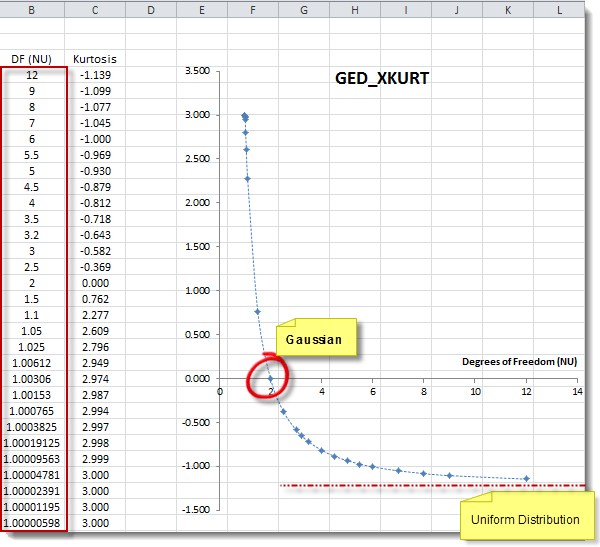# GED_XKURT - GED Excess-Kurtosis

Calculates the excess kurtosis of the generalized error distribution (GED).

## Syntax

GED_XKURT(V)

V
is the shape parameter (or degrees of freedom) of the distribution (V > 1).

## Remarks

1. GED_XKURT is declared as deprecated. Please, use DIST_XKURT as GED_XKURT is listed here for backward compatibility.
2. From time to time, it may be necessary for the definitions of some functions to be altered or removed from the add-in. In these circumstances, the functions will first be declared deprecated and then removed from subsequent versions.
3. The generalized error distribution is also known as the exponential power distribution.
4. The probability density function of the GED is defined as: $$pdf(x)= \frac{e^{-\left |x \right |^\nu}}{2\Gamma(1+\frac{1}{\nu})}$$ Where:
• $\nu$ is the shape parameter (or degrees of freedom).
5. The excess-kurtosis for GED(v) is defined as: $$\gamma_2= \frac{\Gamma (\frac{1}{\nu})\Gamma(\frac{5}{\nu})}{\Gamma(\frac{3}{\nu})^2}-3$$ Where:
• $\Gamma (.)$ is the gamma function.
• $\nu$ is the shape parameter.
6. IMPORTANT The GED excess kurtosis is only defined for shape parameters (degrees of freedom) greater than one.
7. Special Cases:
1. $\nu=2$ GED becomes a normal distribution.
2. $\nu \to \infty$ GED approaches uniform distribution. $$\lim_{\nu \to \infty} \gamma_2(\nu) = -1.2$$
3. $\nu \to 1^+$ GED exhibits the highest excess kurtosis (3). $$\lim_{\nu \to 1^+}\gamma_2(\nu)=3$$

## Examples

Example 1:

Formula Description (Result)
=GED_XKURT(2) GED(2) is Normal distribution (0.000).
=GED_XKURT(1.0001) Maximum excess kurtosis of a GED is 3.0 (3.000).
=GED_XKURT(100) GED approaches uniform distribution for v >> 1 (-1.199).

Example 2:

GED X-Kurtosis Plot